/

### Function Description

Returns an array of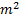elements corresponding to the elements of the matrix,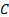, of weighted (Pearson) correlation coefficients for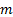series that are provided to the function as an array of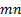elements, where the firstelements correspond to the terms in the first series, the nextelements to the corresponding terms in the second series etc.

Weighted correlation coefficients given weights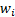(for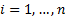), are calculated as: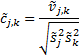where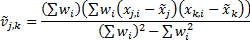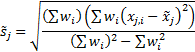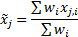See Weighted Moments and Cumulants for further details and explanation of terms in this formula. If the weights are equal then returns the same as MnCorrelations.

Contents | Prev | Next